## Glossary of Scientific Measurements and Laboratory Measurement Tools Terms and Terminology

• The metric system: The internationally agreed to measurement system that is, with the exception of the United States, the only system of measurement that is used.
• International System of Units: Also referred to as SI units, are units of measure approved and supported by the globe
• Base units of measurement: Kilogram, liter for liquid measurements, meter and second,
• Centi: 1/100 or 1 one hundredth
• Deca: 10
• Hecta: 100
• Kilo: 1000
• Mlli: 1 million or 1.000,000
• Mass: The physical property of substances that is the resistance of an object to movement when a force is applied and it is expressed in terms of kilograms (kg).
• Volume: The physical property of substances that is the measurement of the quantity or amount of matter in a three dimensional space.
• Weight: The force of an object against gravity
• Distance: The length of one end to another
• Time: The duration of things and events during the past, present and future.
• Triple Beam Balance Measurement Scale: An instrument that is used to scientifically measure the mass of an object
• Electronic balance scales and simple weighing scales: Instruments that are used to scientifically weigh objects in the context of gravitational force
• Pan balance scales: Scales that operate with a fulcrum and two pans to scientifically weigh objects
• Volumetric flasks: Used for the precise scientific measurement of the volumes of liquids
• Graduated cylinders: Used for the precise scientific measurement of the volumes of liquids
• Volumetric pipettes: Used for the precise scientific measurement of the volumes of liquids.

## The Metric System

The metric system is an internationally agreed to measurement system that is, with the exception of the United States, the only system of measurement that is used.

This system of measurement is used to weights and mass, distance and time. The metric system is based on units of measure approved and supported by the International System of Units, which is referred to as the SI units.

As you will see below, the metric system is built on 10s and multiples, or powers, of tens. More information about the metric system and other systems of measurement was previously detailed in the Mathematics section of this TEAS review under the heading "Measurement and Data".

The base units for weights and mass, volume, distance and time are kilogram, liter for liquid measurements, meter and second, respectively.

As previously discussed:

• Mass is the physical property of substances that is the resistance of an object to movement when a force is applied and it is expressed in terms of kilograms (kg)
• Volume is the physical property of substances that is the measurement of the quantity or amount of matter in a three dimensional space
• Weight is the force of an object against gravity
• Distance is the length of one end to another
• Time is the duration of things and events during the past, present and future.

Measurements less than and more than one unit of measurement for weights and mass, volume, distance and time are preceded with a prefix such as:

• Centi which means 1/100 or 1 one hundredth
• Deca which means 10
• Hecta which means 1 hundred or 100
• Kilo which means 1 thousand or 1000
• Mlli which means 1 million or 1.000,000

For example, a kilometer is 1,000 meters and 1 meter is 1000 millimeters.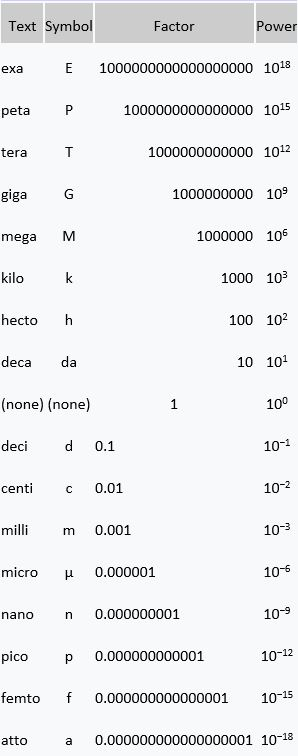Measurements of length which are variations of meter with a prefix include:

• 1 centimeter = 10 millimeters
• 1 decimeter = 10 centimeters or 100 millimeters
• 1,000 millimeters (1 meter) = 100 centimeters
• 1 kilometer = 1,000 meters

## Instruments for Measurement

Instruments that are used to measure mass and weight are:

• Triple beam balance measurement scale
• Electronic balance measurement scale

### Triple Beam Balance Measurement Scales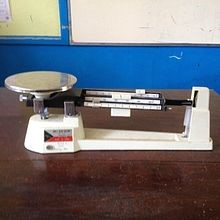A triple beam balance, as shown in the picture above, is an instrument that is used to scientifically measure the mass of an object

### Electronic Balance Measurement Scales

Electronic balance scales and simple weighing scales, as shown in the picture above, weigh objects in the context of gravitational force.

Pan Balance Scales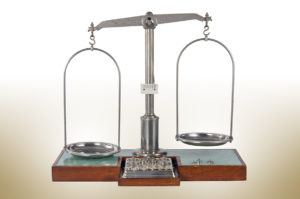Pan balance scales, as shown above, operate with a fulcrum and two pans. One pan is used to hold a known weight object and the other pan is used to hold the object of unknown weight. Known weight objects are added until the scales are balanced. The unknown weight is then determined when the known weights are balanced with the unknown weighted object.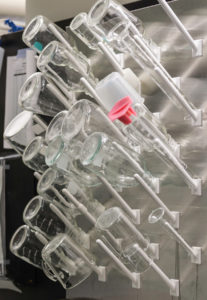Volumetric flasks, as shown in the picture above, are used for the precise scientific measurement of the volumes of liquids.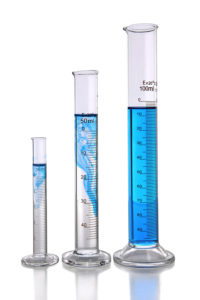Graduated cylinders, as shown in the picture above, are, similar to volumetric flasks; they too are used for the precise scientific measurement of the volumes of liquids.

### Volumetric Pipettes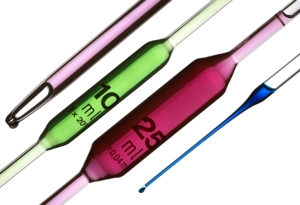Again, volumetric pipettes, as shown in the picture above, are used for the precise scientific measurement of the volumes of liquids.

RELATED TEAS SCIENTIFIC REASONING CONTENTLatest posts by Alene Burke, RN, MSN (see all)